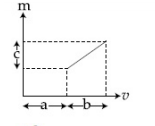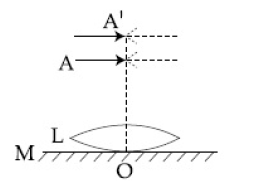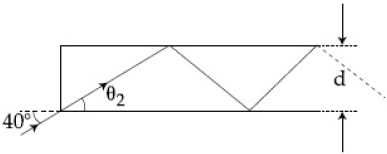## Filters

Sort by :
Clear All
Q

An interference experiment ratio of amplitude of coherent waves is. What is the ratio of maximum and minimum intensities of frings?

• Option 1)

1

• Option 2)

2

• Option 3)

3

• Option 4)

4

Option 1) 1 Option 2) 2 Option 3) 3 Option 4) 4

A concave mirror has radius of curvature of 40 cm. It is at the bottom of a glass that has water filled up to 5 cm (see figure). If a small particle is floating on the surface of water, its image as seen, from directly above the glass, is at a distance d from the surface of water. The value of d is close to :

(Refractive index of water =1.33)• Option 1)

• Option 2)

• Option 3)

• Option 4)

As we can see first the image will be formed by mirror                                Refraction from water surface Option 1) Option 2) Option 3) Option 4)

In a double slit experiment, when a thin film of thickness t having refractive index  is introduced in front of one of the slits, the maximum at the centre of the fringe pattern shifts by one fringe width. The value of t is (  is the wavelenth of the light used) :

• Option 1)

• Option 2)

• Option 3)

• Option 4)

In YDSE Option 1) Option 2) Option 3) Option 4)

In a Young's double slit experiment , the ratio of the slit 's width is 4:1 The ratio of the intensity of maxima to minima , close to the central fringe in the screen , will be:

• Option 1)

• Option 2)

• Option 3)

• Option 4)

Option 1)Option 2)Option 3)Option 4)

The graph shows how the magnification m produced by a thin lens varies with image distance .

What is the focal length of the lens used?• Option 1)

• Option 2)

• Option 3)

• Option 4)

Option 1)            Option 2)Option 3)Option 4)

The value of numerical aperature of the objective lens of a microscope is 1.25. If light of wavelength 5000 A is used, the minimum seperation between two points, to be seen as distinct, will be :

• Option 1)

• Option 2)

• Option 3)

• Option 4)

Resolving power of microscope - -     Minimum distance between two lines    where N.A is numerical opperature   Option 1) Option 2) Option 3) Option 4)

A ray of light AO in vacuum is incident on a glass slab at angle  and

refracted at angle  along OB as shown in the figure. The optical path length of light

ray from A to B is :• Option 1)

• Option 2)

• Option 3)

• Option 4)

Optical Path -   - wherein Distance travelled in vacuum Distance travelled in a medium of refractive index      Optical path = AO +  ( OB ) Apply Snells law , So,  Similarly, =>  =>  So, optical path = AO +  ( OB )                           =                           = 2a+2b        Option 1) Option 2) Option 3) Option 4)

One plano-convex and one plano-concave lens of same radius of curvature 'R' but of

different materials are joined side by side as shown in the figure. If the refractive index of the material

of 1 is  and that of 2 is  , then the focal length of the combination is:• Option 1)

• Option 2)

• Option 3)

• Option 4)

Lensmaker's Formula -   - wherein refractive index of medium of object refractive index of lens are radius of curvature of two surface     Lens placed close to each other - Equivalent focal length.   - wherein are focal lenght of lenc 1, 2, 3, -----n     For lens 1 ..................(1) For lens 2 ..................(2)           Option 1) Option 2) Option 3) Option 4)

A system of three polarizers  is set up such that the pass axis of  is crossed with respect to that of . The pass axis of  is inclined at  to the pass axis of . When a beam of unpolarized light of intensity  is incident on , the intensity of light transmitted by the three polarizers is I. The ratio  equal (nearly):

• Option 1)

5.33

• Option 2)

16.00

• Option 3)

10.67

• Option 4)

1.80

Malus Law - angle made by E vector with transmission axis. - wherein Intensity of transmitted light after polarisation . Intensity of  incident light.     the intensity of light transmitted from  the intensity of light transmitted from  The intensity of light transmitted from     Option 1) 5.33 Option 2) 16.00 Option 3) 10.67 Option 4) 1.80

Calculate the limit of resolution of a telescope objective having a diameter of 200 cm, if it has to detect light of wavelength 500 nm coming from a star.

• Option 1)

• Option 2)

• Option 3)

• Option 4)

Limit of resolution of telescope Option 1)Option 2)Option 3)Option 4)

A convex lens (of focal length 20 cm) and a concave mirror, having their principal axis along the same lines, are kept 80 cm apart from each other. the concave mirror is to the right of the convex lens, its image remains at the same position even if the concave mirror is removed. The maximum distance of the object for which this concave mirror, by itself would produce a virtual image would be:

• Option 1)

30 cm

• Option 2)

25 cm

• Option 3)

10 cm

• Option 4)

20 cm

using lens formula distance of this Image from mirror is 20 cm. As per given condition Image should be form, at centre of circle of mirror  so,  Thus , Maximum distance =10 cmOption 1)30 cmOption 2)25 cmOption 3)10 cmOption 4)20 cm

A transparent cube of side d, made of a material of refractive index  , is immersed in a liquid of refractive index . A ray is incident on the face AB at an angle  (shown in the figure). Total internal reflection takes place at point E on the face BC.Then  must satisfy:

• Option 1)

• Option 2)

• Option 3)

• Option 4)

Option 1)     Option 2) Option 3) Option 4)

A thin convex lens L ( refractive index =   ) is placed on a plane mirror M. When a pin is placed at A , such that  , its real inverted image is formed at A itself , as shown in figure . When a liquid of refractive index  is put between the lens and the mirror , the pin has to be moved to A' such that  , to get its inverted real image at A' itself . The value of  will be :• Option 1)

• Option 2)

• Option 3)

• Option 4)

Option 1) Option 2) Option 3) Option 4)

A convex lens of focal length  produces images of the same magnification  when an object is kept at two distances  from the lens . The ratio of  is :

• Option 1)

• Option 2)

• Option 3)

• Option 4)

By using lens formula Option 1) Option 2) Option 3) Option 4)

Diameter of the objective lens of a telescope is  . For light of wavelength . coming from a distant object , the limit of resolution of the telescope is close to :

• Option 1)

• Option 2)

• Option 3)

• Option 4)

Option 1) Option 2) Option 3) Option 4)

The figure shows a Young's double slit experimental setup. It is observed that when a thin transparent sheet of thickness  and refractive index  is put in front of one of the slits , the central maximum gets shifted by a distance equal to  fringewidths. If the wavelength of lightused is  will be :• Option 1)

• Option 2)

• Option 3)

• Option 4)

If sheet is placed infront of one slit , displacement of fring                   here                 given,                                                      Option 1)                    Option 2) Option 3) Option 4)

A concave mirror for face viewing has focal length of  The distance at which you hold the mirror from your face in order to see your image upright with a magnification of  is :

• Option 1)

• Option 2)

• Option 3)

• Option 4)

Option 1) Option 2)Option 3)Option 4)

An upright object is placed at a distance of 40 cm in front of a convergent lens of focal length 20 cm. A convergent mirror of focal length 10 cm is placed at a distance of 60 cm on the other side of the lens. The position and size of the final image will be :

• Option 1)

from the convergent mirror, same size as the object

• Option 2)

from the convergent mirror, twice the size of the object

• Option 3)

from the convergent mirror, same size as the object

• Option 4)

from the convergent lens, same size of the object

(1) Refraction from lense and (2) Reflection from mirror and  (3) Refraction from lens and Final image is at from convergent lens and it has same size as object. Option 1) from the convergent mirror, same size as the object   Option 2) from the convergent mirror, twice the size of the object Option 3) from the convergent mirror, same size as the object   Option 4) from the...

In figure, the optical fiber is long and has a diameter of If ray of light is incident on one end of the fiber at angle the number of reflections it makes before emerging from the other end close to :

(refractive index of fiber is and )• Option 1)

• Option 2)

• Option 3)

• Option 4)

Snells law for 1st surface and For T.I.R at 2nd surface and So So T.I.R will happen So So Option 1)Option 2)Option 3)  Option 4)

In an interference experiment the ratio of amplitudes of coherent waves is . The ratio of maximum and minimum intensities of fringes will be :

• Option 1)

4

• Option 2)

18

• Option 3)

2

• Option 4)

9

Option 1) 4 Option 2) 18 Option 3) 2 Option 4) 9
Exams
Articles
Questions